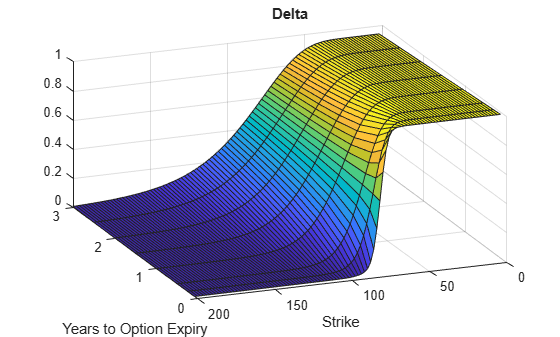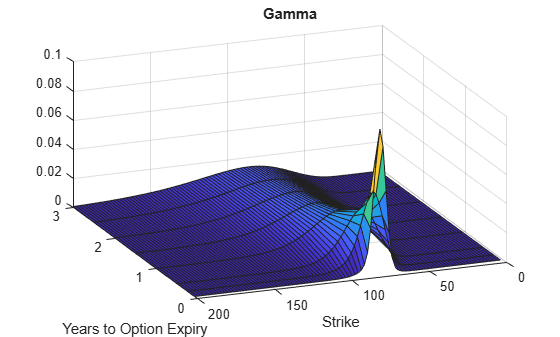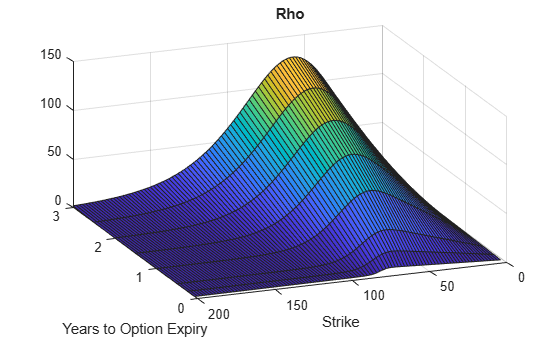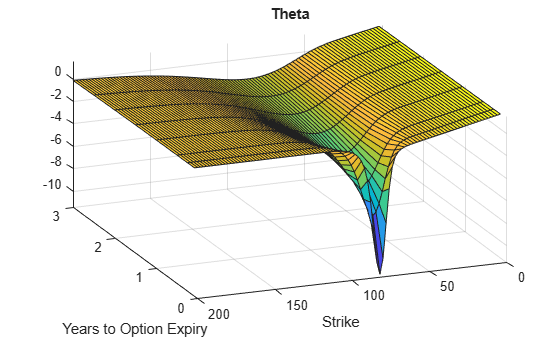# optSensByMertonNI

Option price and sensitivities by Merton76 model using numerical integration

## Syntax

``PriceSens = optSensByMertonNI(Rate,AssetPrice,Settle,Maturity,OptSpec,Strike,Sigma,MeanJ,JumpVol,JumpFreq)``
``PriceSens = optSensByMertonNI(___,Name,Value)``

## Description

example

````PriceSens = optSensByMertonNI(Rate,AssetPrice,Settle,Maturity,OptSpec,Strike,Sigma,MeanJ,JumpVol,JumpFreq)` computes vanilla European option price and sensitivities by the Merton76 model, using numerical integration.```

example

````PriceSens = optSensByMertonNI(___,Name,Value)` adds optional name-value pair arguments. ```

## Examples

collapse all

`optSensByMertonNI` uses numerical integration to compute option sensitivities and then plot option sensitivity surfaces.

Define Option Variables and Merton76 Model Parameters

```AssetPrice = 80; Rate = 0.03; DividendYield = 0.02; OptSpec = 'call'; Sigma = 0.16; MeanJ = 0.02; JumpVol = 0.08; JumpFreq = 2;```

Compute the Option Sensitivity for a Single Strike

```Settle = datenum('29-Jun-2017'); Maturity = datemnth(Settle, 6); Strike = 80; Delta = optSensByMertonNI(Rate, AssetPrice, Settle, Maturity, OptSpec, Strike, ... Sigma, MeanJ, JumpVol, JumpFreq, 'DividendYield', DividendYield, ... 'OutSpec', "delta")```
```Delta = 0.5283 ```

Compute the Option Sensitivities for a Vector of Strikes

The `Strike` input can be a vector.

```Settle = datenum('29-Jun-2017'); Maturity = datemnth(Settle, 6); Strike = (76:2:84)'; Delta = optSensByMertonNI(Rate, AssetPrice, Settle, Maturity, OptSpec, Strike, ... Sigma, MeanJ, JumpVol, JumpFreq, 'DividendYield', DividendYield, ... 'OutSpec', "delta")```
```Delta = 5×1 0.6727 0.6013 0.5283 0.4565 0.3883 ```

Compute the Option Sensitivities for a Vector of Strikes and a Vector of Dates of the Same Lengths

Use the `Strike` input to specify the strikes. Also, the `Maturity` input can be a vector, but it must match the length of the `Strike` vector if the `ExpandOutput` name-value pair argument is not set to `"true"`.

```Settle = datenum('29-Jun-2017'); Maturity = datemnth(Settle, [12 18 24 30 36]); % Five maturities Strike = [76 78 80 82 84]'; % Five strikes Delta = optSensByMertonNI(Rate, AssetPrice, Settle, Maturity, OptSpec, Strike, ... Sigma, MeanJ, JumpVol, JumpFreq, 'DividendYield', DividendYield, ... 'OutSpec', "delta")```
```Delta = 5×1 0.6419 0.5907 0.5565 0.5311 0.5110 ```
` % Five values in vector output`

Expand the Output for a Surface

Set the `ExpandOutput` name-value pair argument to `"true"` to expand the output into a `NStrikes`-by-`NMaturities` matrix. In this case, it is a square matrix.

```Delta = optSensByMertonNI(Rate, AssetPrice, Settle, Maturity, OptSpec, Strike, ... Sigma, MeanJ, JumpVol, JumpFreq, 'DividendYield', DividendYield, ... 'OutSpec', "delta", 'ExpandOutput', true) % (5 x 5) matrix output```
```Delta = 5×5 0.6419 0.6305 0.6245 0.6204 0.6173 0.5922 0.5907 0.5905 0.5905 0.5905 0.5422 0.5507 0.5565 0.5607 0.5637 0.4927 0.5112 0.5229 0.5311 0.5372 0.4447 0.4725 0.4898 0.5020 0.5110 ```

Compute the Option Sensitivities for a Vector of Strikes and a Vector of Dates of Different Lengths

When `ExpandOutput` is `"true"`, `NStrikes` do not have to match `NMaturities`. That is, the output `NStrikes`-by-`NMaturities` matrix can be rectangular.

```Settle = datenum('29-Jun-2017'); Maturity = datemnth(Settle, 12*(0.5:0.5:3)'); % Six maturities Strike = (76:2:84)'; % Five strikes Delta = optSensByMertonNI(Rate, AssetPrice, Settle, Maturity, OptSpec, Strike, ... Sigma, MeanJ, JumpVol, JumpFreq, 'DividendYield', DividendYield, ... 'OutSpec', "delta", 'ExpandOutput', true) % (5 x 6) matrix output```
```Delta = 5×6 0.6727 0.6419 0.6305 0.6245 0.6204 0.6173 0.6013 0.5922 0.5907 0.5905 0.5905 0.5905 0.5283 0.5422 0.5507 0.5565 0.5607 0.5637 0.4565 0.4927 0.5112 0.5229 0.5311 0.5372 0.3883 0.4447 0.4725 0.4898 0.5020 0.5110 ```

Compute the Option Sensitivities for a Vector of Strikes and a Vector of Asset Prices

When `ExpandOutput` is `"true"`, the output can also be a `NStrikes`-by-`NAssetPrices` rectangular matrix by accepting a vector of asset prices.

```Settle = datenum('29-Jun-2017'); Maturity = datemnth(Settle, 12); % Single maturity ManyAssetPrices = [70 75 80 85]; % Four asset prices Strike = (76:2:84)'; % Five strikes Delta = optSensByMertonNI(Rate, ManyAssetPrices, Settle, Maturity, OptSpec, Strike, ... Sigma, MeanJ, JumpVol, JumpFreq, 'DividendYield', DividendYield, ... 'OutSpec', "delta", 'ExpandOutput', true) % (5 x 4) matrix output```
```Delta = 5×4 0.3796 0.5157 0.6419 0.7472 0.3315 0.4637 0.5922 0.7043 0.2874 0.4137 0.5422 0.6592 0.2474 0.3664 0.4927 0.6128 0.2117 0.3224 0.4447 0.5657 ```

Plot Option Sensitivity Surfaces

The `Strike` and `Maturity` inputs can be vectors. Set `ExpandOutput` to `"true"` to output the surfaces as `NStrikes`-by-`NMaturities` matrices.

```Settle = datenum('29-Jun-2017'); Maturity = datemnth(Settle, 12*[1/12 0.25 (0.5:0.5:3)]'); Times = yearfrac(Settle, Maturity); Strike = (2:2:200)'; [Delta, Gamma, Rho, Theta, Vega] = optSensByMertonNI(... Rate, AssetPrice,Settle, Maturity, OptSpec, Strike, ... Sigma, MeanJ, JumpVol, JumpFreq, 'DividendYield', DividendYield, ... 'OutSpec', ["delta", "gamma", "rho", "theta", "vega"], ... 'ExpandOutput', true); [X,Y] = meshgrid(Times,Strike); figure; surf(X,Y,Delta); title('Delta'); xlabel('Years to Option Expiry'); ylabel('Strike'); view(-112,34); xlim([0 Times(end)]);``````figure; surf(X,Y,Gamma) title('Gamma') xlabel('Years to Option Expiry') ylabel('Strike') view(-112,34); xlim([0 Times(end)]);``````figure; surf(X,Y,Rho) title('Rho') xlabel('Years to Option Expiry') ylabel('Strike') view(-112,34); xlim([0 Times(end)]);``````figure; surf(X,Y,Theta) title('Theta') xlabel('Years to Option Expiry') ylabel('Strike') view(-112,34); xlim([0 Times(end)]);``````figure; surf(X,Y,Vega) title('Vega') xlabel('Years to Option Expiry') ylabel('Strike') view(-112,34); xlim([0 Times(end)]);```## Input Arguments

collapse all

Continuously compounded risk-free interest rate, specified as a scalar decimal value.

Data Types: `double`

Current underlying asset price, specified as numeric value using a scalar or a `NINST`-by-`1` or `NColumns`-by-`1` vector.

For more information on the proper dimensions for `AssetPrice`, see the name-value pair argument `ExpandOutput`.

Data Types: `double`

Option settlement date, specified as a `NINST`-by-`1` or `NColumns`-by-`1` vector using serial date numbers, date character vectors, datetime arrays, or string arrays. The `Settle` date must be before the `Maturity` date.

For more information on the proper dimensions for `Settle`, see the name-value pair argument `ExpandOutput`.

Data Types: `double` | `char` | `datetime` | `string`

Option maturity date, specified as a `NINST`-by-`1` or `NColumns`-by-`1` vector using serial date numbers, date character vectors, datetime arrays, or string arrays.

For more information on the proper dimensions for `Maturity`, see the name-value pair argument `ExpandOutput`.

Data Types: `double` | `char` | `datetime` | `string`

Definition of the option, specified as a `NINST`-by-`1` or `NColumns`-by-`1` vector using a cell array of character vectors or string arrays with values `'call'` or `'put'`.

For more information on the proper dimensions for `OptSpec`, see the name-value pair argument `ExpandOutput`.

Data Types: `cell` | `string`

Option strike price value, specified as a `NINST`-by-`1`, `NRows`-by-`1`, `NRows`-by-`NColumns` vector of strike prices.

For more information on the proper dimensions for `Strike`, see the name-value pair argument `ExpandOutput`.

Data Types: `double`

Volatility of the underling asset, specified as a scalar numeric value.

Data Types: `double`

Mean of the random percentage jump size (J), specified as a scalar decimal value where `log`(1+J) is normally distributed with mean (`log`(1+`MeanJ`)-0.5*`JumpVol`^2) and the standard deviation `JumpVol`.

Data Types: `double`

Standard deviation of `log`(1+J) where `J` is the random percentage jump size, specified as a scalar decimal value.

Data Types: `double`

Annual frequency of Poisson jump process, specified as a scalar numeric value.

Data Types: `double`

### Name-Value Arguments

Specify optional pairs of arguments as `Name1=Value1,...,NameN=ValueN`, where `Name` is the argument name and `Value` is the corresponding value. Name-value arguments must appear after other arguments, but the order of the pairs does not matter.

Before R2021a, use commas to separate each name and value, and enclose `Name` in quotes.

Example: ```Price = optSensByMertonNI(Rate,AssetPrice,Settle,Maturity,OptSpec,Strike,Sigma,MeanJ,JumpVol,JumpFreq,'Basis',7)```

Day-count of the instrument, specified as the comma-separated pair consisting of `'Basis'` and a scalar using a supported value:

• 0 = actual/actual

• 1 = 30/360 (SIA)

• 2 = actual/360

• 3 = actual/365

• 4 = 30/360 (PSA)

• 5 = 30/360 (ISDA)

• 6 = 30/360 (European)

• 7 = actual/365 (Japanese)

• 8 = actual/actual (ICMA)

• 9 = actual/360 (ICMA)

• 10 = actual/365 (ICMA)

• 11 = 30/360E (ICMA)

• 12 = actual/365 (ISDA)

• 13 = BUS/252

Data Types: `double`

Continuously compounded underlying asset yield, specified as the comma-separated pair consisting of `'DividendYield'` and a scalar numeric value.

Data Types: `double`

Define outputs, specified as the comma-separated pair consisting of `'OutSpec'` and a `NOUT`- by-`1` or a `1`-by-`NOUT` string array or cell array of character vectors with supported values.

Note

`"vega"` is the sensitivity with respect the initial volatility sqrt(`V0`).

Example: ```OutSpec = ["price","delta","gamma","vega","rho","theta"]```

Data Types: `string` | `cell`

Absolute error tolerance for numerical integration, specified as the comma-separated pair consisting of `'AbsTol'` and a scalar numeric value.

Data Types: `double`

Relative error tolerance for numerical integration, specified as the comma-separated pair consisting of `'RelTol'` and a scalar numeric value.

Data Types: `double`

Numerical integration range used to approximate the continuous integral over `[0 Inf]`, specified as the comma-separated pair consisting of `'IntegrationRange'` and a `1`-by-```2 ```vector representing `[LowerLimit UpperLimit]`.

Data Types: `double`

Framework for computing option prices and sensitivities using numerical integration of models, specified as the comma-separated pair consisting of `'Framework'` and a scalar string or character vector with the following values:

• `"heston1993"` or `'heston1993'` — Method used in Heston (1993)

• `"lewis2001"` or `'lewis2001'` — Method used in Lewis (2001)

Data Types: `char` | `string`

Flag to expand the outputs, specified as the comma-separated pair consisting of `'ExpandOutput'` and a logical:

• `true` — If `true`, the outputs are `NRows`-by- `NColumns` matrices. `NRows` is the number of strikes for each column and it is determined by the `Strike` input. For example, `Strike` can be a `NRows`-by-`1` vector, or a `NRows`-by-`NColumns` matrix. `NColumns` is determined by the sizes of `AssetPrice`, `Settle`, `Maturity`, and `OptSpec`, which must all be either scalar or `NColumns`-by-`1` vectors.

• `false` — If `false`, the outputs are `NINST`-by-`1` vectors. Also, the inputs `Strike`, `AssetPrice`, `Settle`, `Maturity`, and `OptSpec` must all be either scalar or `NINST`-by-`1` vectors.

Data Types: `logical`

## Output Arguments

collapse all

Option prices or sensitivities, returned as a `NINST`-by-`1`, or `NRows`-by-`NColumns`, depending on `ExpandOutput`. The name-value pair argument `OutSpec` determines the types and order of the outputs.

collapse all

### Vanilla Option

A vanilla option is a category of options that includes only the most standard components.

A vanilla option has an expiration date and straightforward strike price. American-style options and European-style options are both categorized as vanilla options.

The payoff for a vanilla option is as follows:

• For a call: $\mathrm{max}\left(St-K,0\right)$

• For a put: $\mathrm{max}\left(K-St,0\right)$

where:

St is the price of the underlying asset at time t.

K is the strike price.

### Merton Jump Diffusion Model

The Merton jump diffusion model (Merton (1976)) is a different extension of the Black-Scholes model, where sudden asset price movements (both up and down) are modeled by adding the jump diffusion parameters with the Poisson process.

The stochastic differential equation is:

`$\begin{array}{l}d{S}_{t}=\left(r-q-{\lambda }_{p}{\mu }_{j}\right){S}_{t}dt+\sigma {S}_{t}d{W}_{t}+J{S}_{t}d{P}_{t}\\ \text{prob(}d{P}_{t}=1\right)={\lambda }_{p}dt\end{array}$`

where

r is the continuous risk-free rate.

q is the continuous dividend yield.

Wt is the Weiner process.

J is the random percentage jump size conditional on the jump occurring, where `ln`(1+J) is normally distributed with mean $\mathrm{ln}\left(1+{\mu }_{J}\right)-\frac{{\delta }^{2}}{2}$ and the standard deviation δ, and (1+J) has a lognormal distribution:

`$\frac{1}{\left(1+J\right)\delta \sqrt{2\pi }}\mathrm{exp}\left\{{\frac{-\left[\mathrm{ln}\left(1+J\right)-\left(\mathrm{ln}\left(1+{\mu }_{J}\right)-\frac{{\delta }^{2}}{2}\right]}{2{\delta }^{2}}}^{2}\right\}$`

μJ is the mean of J for (μJ > -1).

δ is the standard deviation of `ln`(1+J) for (δ≥ 0).

ƛp is the annual frequency (intensity) of Poisson process Ptfor (ƛp ≥ 0).

σ is the volatility of the asset price for (σ > 0).

The characteristic function ${f}_{Merton{76}_{j}}\left(\varphi \right)$ for j = 1 (asset prices measure) and j = 2 (risk-neutral measure) is:

where

ϕ is the characteristic function variable.

τ is the time to maturity (τ = T- t).

i is the unit imaginary number ( i2 = -1).

### Numerical Integration Method Under Heston (1993) Framework

Numerical integration is used to evaluate the continuous integral for the inverse Fourier transform.

The numerical integration method under the Heston (1993) framework is based on the following expressions:

`$\begin{array}{l}Call\left(K\right)={S}_{t}{e}^{-q\tau }{P}_{1}-K{e}^{-r\tau }{P}_{2}\\ Put\left(K\right)=Call\left(K\right)+K{e}^{-r\tau }-{S}_{t}{e}^{-q\tau }\\ {P}_{j}=\frac{1}{2}+\frac{1}{\pi }\underset{0}{\overset{\infty }{\int }}\mathrm{Re}\left[\frac{{e}^{-i\varphi \mathrm{ln}\left(K\right)}{f}_{j}\left(\varphi \right)}{i\varphi }\right]d\varphi \end{array}$`

where

r is the continuous risk-free rate.

q is the continuous dividend yield.

St is the asset price at time t.

K is the strike.

τ is time to maturity (τ = T-t).

Call(K) is the call price at strike K.

Put(K) is the put price at strike K.

i is a unit imaginary number (i2= -1).

ϕ is the characteristic function variable.

fj(ϕ) is the characteristic function for Pj(j = 1,2).

P1 is the probability of St > K under the asset price measure for the model.

P2 is the probability of St > K under the risk-neutral measure for the model.

Where j = 1,2 so that f1(ϕ) and f2(ϕ) are the characteristic functions for probabilities P1 and P2, respectively.

This framework is chosen with the default value `“Heston1993”` for the `Framework` name-value pair argument.

### Numerical Integration Method Under Lewis (2001) Framework

Numerical integration is used to evaluate the continuous integral for the inverse Fourier transform.

The numerical integration method under the Lewis (2001) framework is based on the following expressions:

`$\begin{array}{l}Call\left(k\right)={S}_{t}{e}^{-q\tau }-\frac{\sqrt{K}{e}^{-\tau t}}{\pi }\underset{0}{\overset{\infty }{\int }}\mathrm{Re}\left[{K}^{-iu}{f}_{2}\left(\varphi =\left(u-\frac{i}{2}\right)\right)\frac{1}{{u}^{2}+\frac{1}{4}}\right]du\\ Put\left(K\right)=Call\left(K\right)=K{e}^{-\tau t}-{S}_{t}{e}^{-q\tau }\end{array}$`

where

r is the continuous risk-free rate.

q is the continuous dividend yield.

St is the asset price at time t.

K is the strike.

τ is time to maturity (τ = T-t).

Call(K) is the call price at strike K.

Put(K) is the put price at strike K.

i is a unit imaginary number (i2= -1).

ϕ is the characteristic function variable.

u is the characteristic function variable for integration, where $\varphi =\left(u-\frac{i}{2}\right)$.

f2(ϕ) is the characteristic function for P2.

P2 is the probability of St > K under the risk-neutral measure for the model.

This framework is chosen with the value `“Lewis2001”` for the `Framework` name-value pair argument.

 Bates, D. S. “Jumps and Stochastic Volatility: Exchange Rate Processes Implicit in Deutsche Mark Options.” The Review of Financial Studies. Vol 9. No. 1. 1996.

 Cont, R. and P. Tankov. Financial Modeling with Jump Processes. Chapman & Hall/CRC Press, 2004.

 Heston, S. L. “A Closed-Form Solution for Options with Stochastic Volatility with Applications to Bond and Currency Options.” The Review of Financial Studies. Vol 6. No. 2. 1993.

 Lewis, A. L. “A Simple Option Formula for General Jump-Diffusion and Other Exponential Levy Processes.” Envision Financial Systems and OptionCity.net, 2001.

 Merton, R. “Option Pricing When Underlying Stock Returns are Discontinuous.” Journal of Financial Economics. Vol 3. 1976.